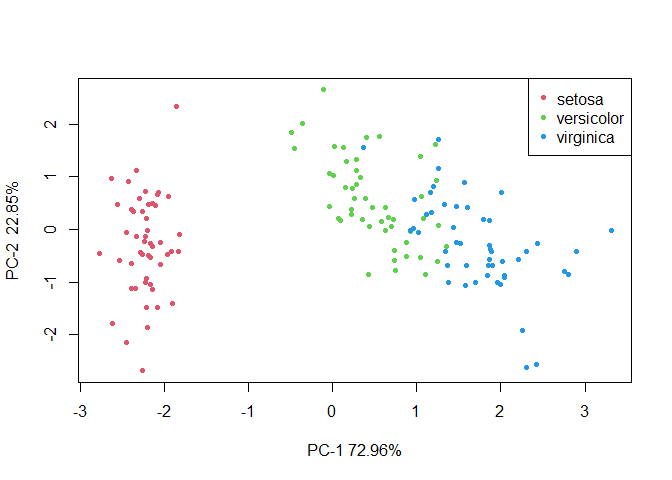# The method

lpda is an R package that addresses the classification problem through linear programming. The method looks for a hyperplane, H, which separates the samples into two groups by minimizing the sum of all the distances to the subspace assigned to the group each individual belongs to. It results in a convex optimization problem for which we find an equivalent linear programming problem. We demonstrated that H exists when the centroids of the two groups are not equal . The method has been extended to more than two groups by considering pairwise comparisons. Moreover, lpda offers the possibility of dealing with Principal Components (PCs) to reduce the dimension of the data avoiding overfitting problems. This option can be applied independently of the number of samples, $$n$$, and variables, $$p$$, that is $$n>p$$ or $$n<p$$. Compared to other similar techniques it is very fast, mainly because it is based in a linear programming problem .

# The package

library(lpda)

Main function is lpda that collect the input data, standarises the data or applies Principal Component Analysis (PCA) through lpda.pca if it is required. Then, it calls to lpda.fit as many times as pairwise comparisons there are. The result is a lpda type object that is the input to compute predictions through ‘predict’ function and to visualize results through ‘plot’ function.

The package has also three functions to compute by crossvalidation (CV) the classification error in different test data sets. This is helpful to decide an appropriate number of PCs or a specific strategy to compute the hyperplane. The functions are:

• bestPC: computes the classification error rate for lpda.pca models obtained with the number of components specified in PCs argument. The result is the average classification error rate from the models computed for each number of PCs.

• bestVariability: computes the classification error rate for lpda.pca models obtained with the number of components needed to reach the explained variability specified in Vars argument. The result is the average classification error rate from the models computed for each explained variability specified in Vars.

• lpdaCV: Computes the classification error rate for a specific model. The user can choose leaf one out (loo) CV, that uses CVloo function, or random test sets with a specified size with ktest option, that uses CVktest function.

# The data

lpda package includes two data sets concerning data science: palmdates and RNAseq. The first one is a real data set from a chemometric study and the second one a simulated RNA-seq experiment. In this document we show the performance of the package with these data sets and with iris data, available in R package.

## Palmdates description

Palmdates is a data set with scores of 21 palm dates including their respective Raman spectra and the concentration of five compounds covering a wide range of concentrations: fibre, glucose, fructose, sorbitol and myo-inositol . The first 11 dates are Spanish (from Elche, Alicante) with no well-defined variety and the last 10 are from other countries and varieties, mainly Arabian. The data set has two data.frames: conc with 5 variables and spectra with 2050.

data("palmdates")
names(palmdates)
#>  "conc"    "spectra"
dim(palmdates$spectra) #>  21 2050 names(palmdates$conc)
#>  "fibre"        "sorbitol"     "fructose"     "glucose"      "myo-inositol"

As conc and spectra, are very correlated, the application of the method with PCs reduces substantially the dimension.

## RNAseq description

This data set has been simulated as Negative Binomial distributed and transformed to rpkm (Reads per kilo base per million mapped reads). It contains 600 genes (in columns) and 60 samples (rows), 30 of each one of the experimental groups. First 30 samples are from first group and the remaining samples from the second one. It has been simulated with few variables (genes) that discriminate between groups. There is few correlation and a lot of noise.

data("RNAseq")
dim(RNAseq)
#>   60 600
#>         Gene1 Gene2 Gene3 Gene4 Gene5 Gene6
#> G1.T1.1    82   154    44    82    55    55
#> G1.T1.2    66    66    15    25    66   112
#> G1.T1.3   141    19   160   175    78    68
#> G1.T1.4   381    57    71   119   186    62
#> G1.T1.5    60    23    88   134   143    60
#> G1.T1.6   149    37    64    16    90   117

# Example 1: Palmdates data

## Chemical variables

First we apply the method with the first two variables: fibre and sorbitol to show the performance of the package. The application of the method with two variables allows the visualization of the hyperplane in two dimensions, in this case, a straight line.

   group = as.factor( c(rep("Spanish",11), rep("Other",10)) )
model1 = lpda(data = palmdates$conc[,1:2], group = group ) First output of lpda is a matrix with the coefficients of the hyperplane: $$a'x=b$$ for each pair-wise comparison. In this example: model1$coef
#>      Other-Spanish
#> [1,]     -1.116131
#> [2,]     -3.002923
#> [3,]     -6.563646

being -1.116 and -3.003 the coefficients of fibre and sorbitol respectively and -6.564 the constant $$b$$. We can plot the line on the points, that represent the samples, with the following code:

   plot(palmdates$conc[,1:2], col = as.numeric(group)+1, pch = 20, main = "Palmdates example") abline(model1$coef/model1$coef, -model1$coef/model1$coef, cex = 2) legend("bottomright", c("Other","Spanish"),col = c(2,3), pch = 20, cex=0.8)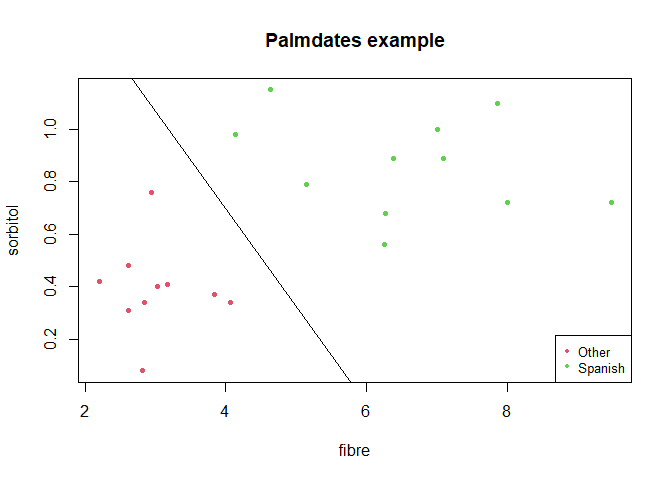Predicted group with the model is obtained with predict function. Confusion matrix is computed as follows. We observe that all the samples are well classified. pred = predict(model1) table(pred$fitted, group)
#>          group
#>           Other Spanish
#>   Other      10       0
#>   Spanish     0      11

By considering the five concentration variables, all samples are well classified as well. As a 5-dimensional plot can not be showed, we offer the possibility of seeing a plot that shows the situation of samples with respect to $$H$$. Y-axis represents order in which they appear in the data matrix and X-axis distances of each sample to $$H$$.

   model2 = lpda(data = palmdates$conc, group = group ) plot(model2)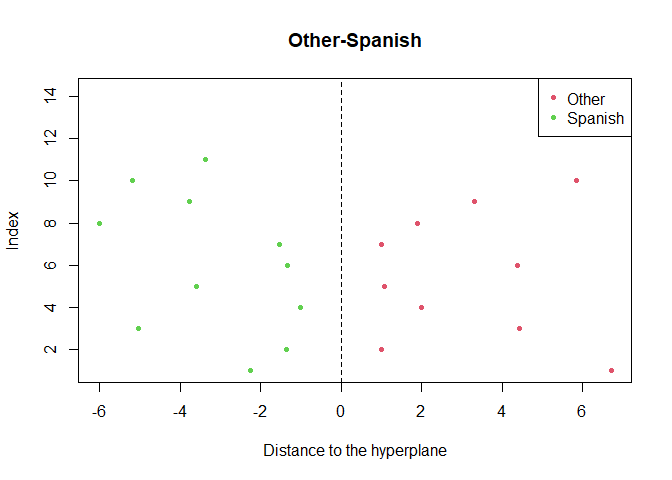Another option is the application of lpda to the first PCs scores. When data is highly correlated, two components are usually preferred. In such case choosing as plot arguments PCscores = TRUE we will visualize the first two PCs, indicating in the axis the proportion of explained variance by each PC. Moreover if there are two groups, as in this example, the optimal hyperplane is also showed. model3 = lpda(data = palmdates$conc, group = group, pca = TRUE, Variability = 0.7)
plot(model3, PCscores = TRUE, main = "PCA-Substances palmdates")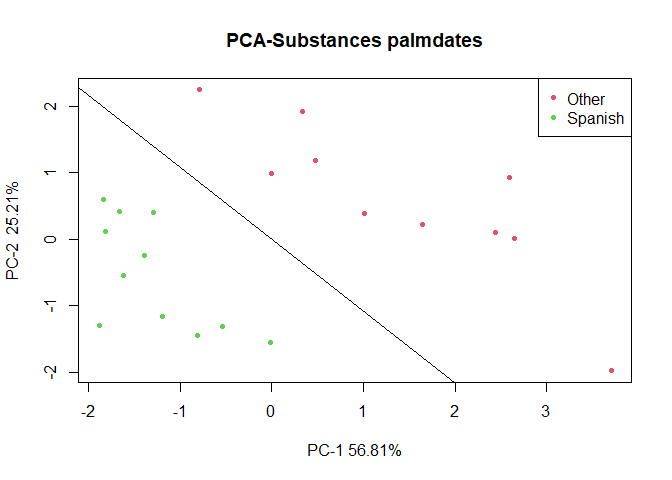## Spectra variables

When having data sets with more variables than individuals PCA is not directly applicable. In lpda we have implemented the possibility of dealing with such problem, working with the equivalences between the PCA of the data matrix and the transposed matrix.

In palmdates$spectra there are 2050 very correlated measurements as it is showed in the following figure: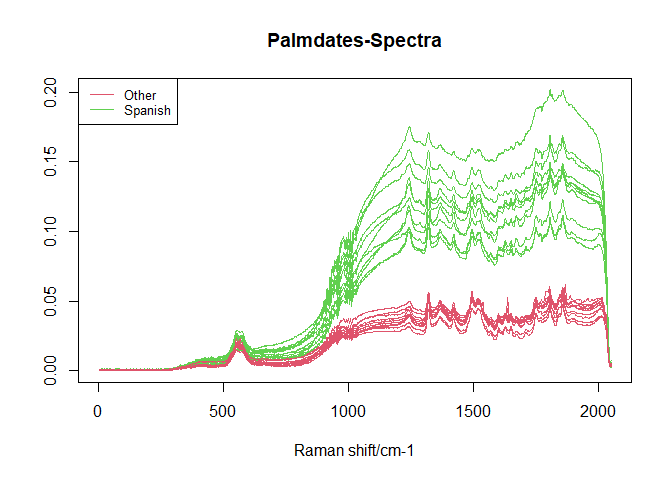Due to the high correlation the application of lpda to all the spectra variables and to the first 2 PCs gives the same solution: 0 prediction errors. model4 <- lpda(data = palmdates$spectra, group = group)
pred = predict(model4)
table(pred$fitted, group) #> group #> Other Spanish #> Other 10 0 #> Spanish 0 11 model5 = lpda(data = palmdates$spectra, group = group, pca = TRUE, Variability = 0.9)
plot(model5, PCscores = TRUE, main = "Spectra palmdates")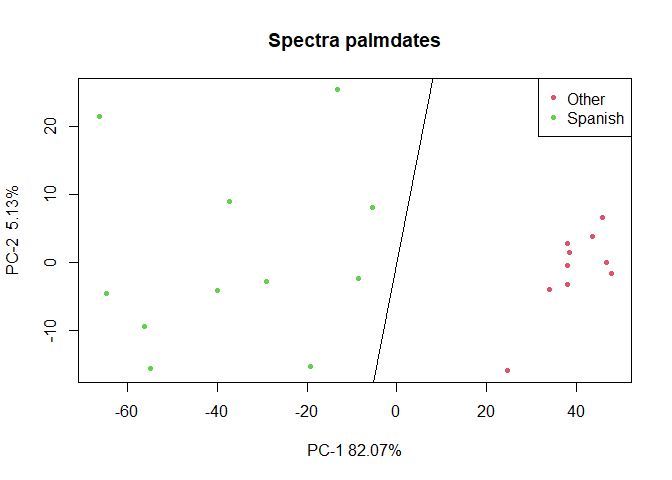Following example shows how predict the group of a test set that does not participate in the model. In this set we include two samples of each group.

test = c(10,11,12,13)
model6 = lpda(data = palmdates$spectra[-test,], group = group[-test], pca = TRUE, Variability = 0.9) pred = predict(model6, palmdates$spectra[test,])
pred$fitted #>  "Spanish" "Spanish" "Other" "Other" We can also use lpdaCV function to compute the predicted error in different test sets by crossvalidation with a specific model. As explained before, two strategies are implemented: loo (leave one out) and ktest that is specified in CV argument and by default is loo. When ktest is selected, ntest is the size of test set (samples not used for computing the model, only to evaluate the number of prediction errors) and R is the times the model is computed and evaluated with different training and test sets. lpdaCV(palmdates$spectra, group, pca = TRUE, CV = "loo")
lpdaCV(palmdates$spectra, group, pca = TRUE, CV = "ktest", ntest = 5, R = 10) CV results are so good due to the clear difference between the two groups in spectra data. We continue with CV functions in Example 2 that deals noisier data. # Example 2: RNAseq simulated data This section applies cros-validation functions with RNAseq data that is noisier than palmdates data and has 60 samples. Firstly we can see that the model with all the data gets a separating hyperplane.  group = as.factor(rep(c("G1","G2"), each = 30)) model = lpda(RNAseq, group) # model with all the variables pred = predict(model) table(pred$fitted, group)
#>     group
#>      G1 G2
#>   G1 30  0
#>   G2  0 30

Evaluating the error in several test sets with CV functions we can see that, to avoid overfitting, it is preferable the application of lpda with PCA:

lpdaCV(RNAseq, group, pca = FALSE, CV = "ktest", ntest = 10)
#>  "Prediction error rate: 0.39"
lpdaCV(RNAseq, group, pca = TRUE, CV = "ktest", ntest = 10)
#>  "Prediction error rate: 0.06"

However, the success of PCA solution depends on the chosen number of components. For this reason it is recommended the application of bestVariability or bestPC functions, that can help to decide the number of PCs that better fit the data.

bestVariability(RNAseq, group, ntest = 10, R = 10, Vars = c(0.1, 0.9))
#>  0.1  0.9
#> 0.09 0.04
bestPC(RNAseq, group, ntest = 10, R = 10, PCs = c(2, 10))
#>    2   10
#> 0.06 0.05

Therefore variability = 0.9 or PC=10 are preferable and the model for future predictions will be:

lpda(RNAseq, group, pca = TRUE, Variability = 0.9)

# Example 3: iris data

To show an example with more than two groups we use the famous (Fisher’s or Anderson’s) iris data set that is available in base R. The iris data set gives the measurements in centimeters of the variables sepal length and width and petal length and width, respectively, for 50 flowers from each of 3 species of iris. The species are Iris setosa, versicolor, and virginica.

Results with the four variables give 2 classification errors. In this case the model computes 3 hyperplanes for each one of the 3 pairwise comparisons. Now plot function gives 3 plots.

model.iris = lpda(iris[,-5], iris[,5])
pred.iris = predict(model.iris)
table(pred.iris$fitted, iris[,5]) #> #> setosa versicolor virginica #> setosa 50 0 0 #> versicolor 0 49 1 #> virginica 0 1 49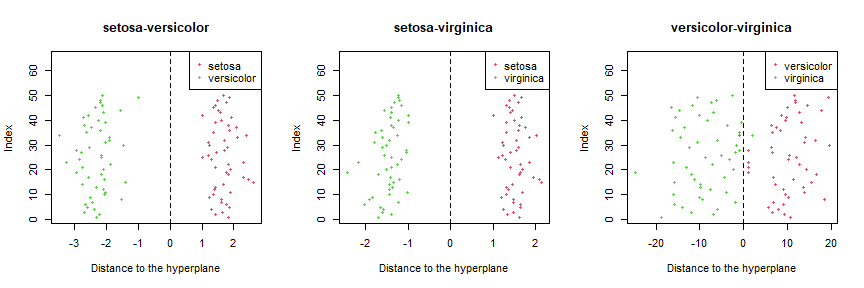The application of lpda with 3 PCs gives the same classification error. Function plot with Pcscores = TRUE gives the scores in the first two PCs, but in this case without the hyperplanes. model.iris2 = lpda(iris[,-5], iris[,5], pca=TRUE, PC=3) pred.iris2 = predict(model.iris2) table(pred.iris2$fitted, iris[,5])
#>
#>              setosa versicolor virginica
#>   setosa         50          0         0
#>   versicolor      0         49         1
#>   virginica       0          1        49
plot(model.iris2, PCscores= TRUE)